#### 期刊菜单

Algorithm on Surface Defect Detection of Strip Based on Improved FCOS
DOI: 10.12677/AIRR.2022.111001, PDF, HTML, XML, 下载: 166  浏览: 345

Abstract: Aiming at the defects of low detection efficiency and limited applicable scope in strip surface defect detection, a steel strip surface defect detection algorithm based on improved FCOS was proposed. The algorithm uses convolutional neural network with deformable convolution to extract defect features, uses key point feature fusion to enhance the detection model input and uses the central point sampling strategy to select training samples to optimize model training. Finally, the proposed algorithm is trained and evaluated on public dataset NEU-DET, Northeastern University surface defect database. On NEU-DET dataset, the mean average precision of this algorithm achieves 74% and the detection velocity is 31.4 FPS.

1. 引言

2. FCOS模型结构

FCOS  模型结构如图1所示，首先使用深度卷积神经网络提取输入图像特征图，然后使用分类网络、回归网络、中心度网络对特征图上的所有特征点逐个进行检测，分类网络输出原图上以此特征点为中心的区域所含缺陷类别，回归网络输出原图上以此特征点为中心的缺陷区域，中心度网络输出特征点在原图上对应的点为中心点的概率，抑制偏心度较大的低质量检测框的输出。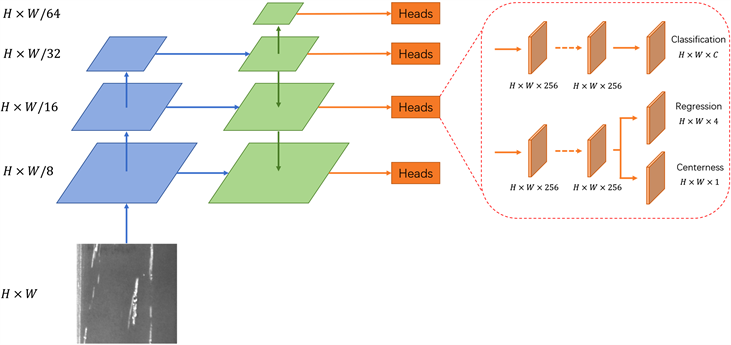Figure 1. The basic structure of improved FCOS model

${F}_{i}\in {ℝ}^{H×W×C}$ 是第i层的特征图，s为该层总的下采样倍率，输入图像中的真实检测框定义为 $\left\{{B}_{i}\right\}$ ，其中 ${B}_{i}=\left({x}_{0}^{\left(i\right)},{y}_{0}^{\left(i\right)},{x}_{1}^{\left(i\right)},{y}_{1}^{\left(i\right)},{c}^{\left(i\right)}\right)\in {ℝ}^{4}×\left\{1,2,\cdots ,C\right\}$ ，此处 $\left({x}_{0}^{\left(i\right)},{y}_{0}^{\left(i\right)}\right)$$\left({x}_{1}^{\left(i\right)},{y}_{1}^{\left(i\right)}\right)$ 分别为真实检测框的左上角点和右下角点的坐标， ${c}^{\left(i\right)}$ 为真实检测框中缺陷的类别，C为缺陷类别个数。对于特征图 ${F}_{i}$ 上的每个位置

$\left(x,y\right)$ ，其在输入图像上的映射位置约为 $\left(⌊\frac{s}{2}⌋+xs,⌊\frac{s}{2}⌋+ys\right)$ ，与Anchor-based通过回归基于此处预设的

$centernes{s}^{*}=\sqrt{\frac{\mathrm{min}\left({l}^{*},{r}^{*}\right)}{\mathrm{max}\left({l}^{*},{r}^{*}\right)}×\frac{\mathrm{min}\left({t}^{*},{b}^{*}\right)}{\mathrm{max}\left({t}^{*},{b}^{*}\right)}}$ (1)

3. FCOS的改进

3.1. 形变卷积

CNN网络由卷积层和池化层组成，由于卷积层和池化层都是对固定尺度的区域进行采样，即在同一层的CNN模块的每个激活单元拥有固定尺度和形状的感受野，然而不同位置的物体的尺度和形状都是不一样的，对于尺度、形状和感受野不匹配的物体，感受野固定的CNN无法充分提取物体的特征(如图2所示)。因此，本文引入形变卷积(Deformable Convolution Network, DCN)  模块增强深度卷积网络特征提取能力。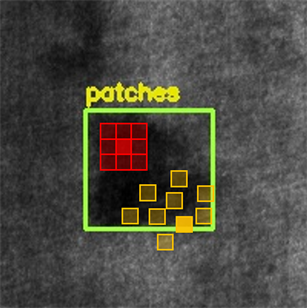Figure 2. 3 × 3 convolution receptive field (the red part is the receptive field of conventional convolution, and the yellow part is the receptive field of deformable convolution)

$y\left({p}_{0}\right)=\underset{{p}_{n}\in \mathcal{R}}{\sum }w\left({p}_{n}\right)\cdot x\left({p}_{0}+{p}_{n}\right)$ (2)

$y\left({p}_{0}\right)=\underset{{p}_{n}\in \mathcal{R}}{\sum }w\left({p}_{n}\right)\cdot x\left({p}_{0}+{p}_{n}+\Delta {p}_{n}\right)$ (3)

$x\left(p\right)=\underset{q}{\sum }G\left(q,p\right)\cdot x\left(q\right),\text{\hspace{0.17em}}\text{\hspace{0.17em}}p={p}_{0}+{p}_{n}+\Delta {p}_{n}$ (4)

$G\left(q,p\right)=g\left({q}_{x},{p}_{x}\right)\cdot g\left({q}_{y},{p}_{y}\right),\text{\hspace{0.17em}}\text{\hspace{0.17em}}g\left(a,b\right)=\mathrm{max}\left(0,1-|a-b|\right)$ (5)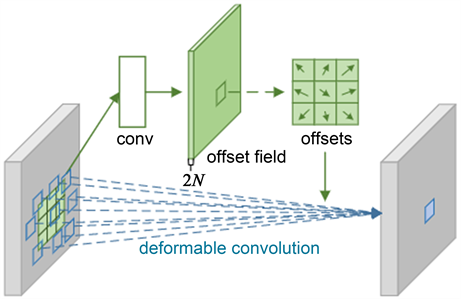Figure 3. Deformable convolution network

3.2. 关键点特征融合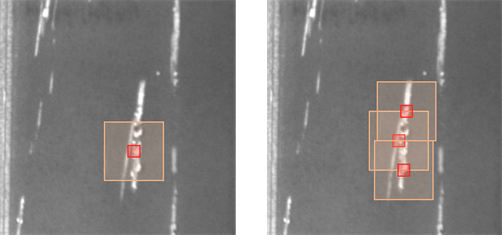Figure 4. Sketch map of single feature point representation and key point set representation (red rectangle is the feature point, and yellow rectangle is the receptive field corresponding to the feature point)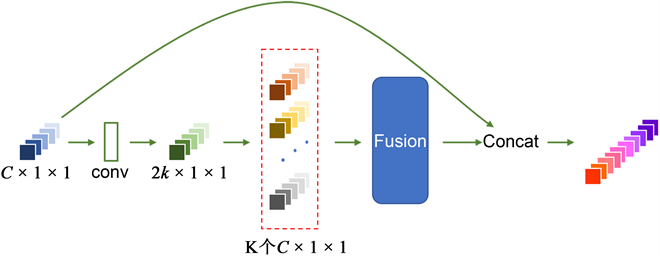Figure 5. Generation and fusion of key point sets

3.3. 中心采样策略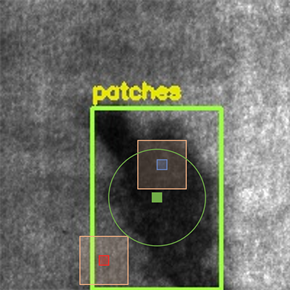Figure 6. Center sampling (the feature points falling in the green ring are regarded as positive samples, and vice versa)

4. 实验结果与分析

4.1. NEU-DET数据集

NEU-DET数据集是东北大学宋克臣团队  制作的钢带表面缺陷数据集，包含6种缺陷类别，分别为裂纹(Crazing)，夹杂(Inclusion)，斑块(Patch)，点蚀表面(Pitted Surface)，轧制氧化皮(Rolled-in Scale)，划痕(Scratch)，每种缺陷含有300张分辨率为200 × 200的灰度图片，总样本数为1800。数据集同时提供每张图片所对应的缺陷标注信息的XML文件，标注信息包括缺陷所属类别与边界框的信息(矩形框左上角和右下角的坐标信息)，共计4189个边界框。图7为NEU-DET数据集中6种表面缺陷图像的示例样本。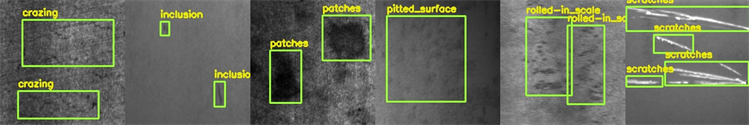Figure 7. Examples of defect sample in NEU-DET dataset

4.2. 评价指标

4.3. 实验结果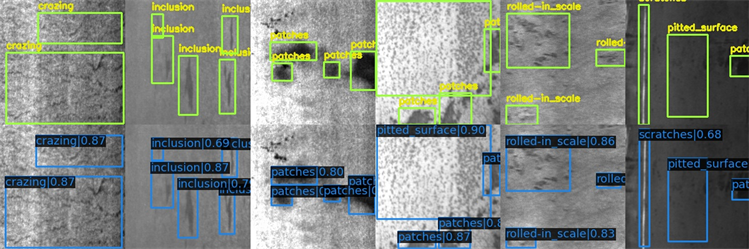Figure 8. Detection results of improved FCOS (top row is ground truth, bottom row is the results of improved FCOS)Table 1. Comparison of experimental results of various defect detection models

5. 总结

  吴平川, 路同浚. 钢板表面缺陷的无损检测技术与应用[J]. 无损检测, 2000, 22(7): 312-315.  顾佳晨, 高雷, 刘路硌. 基于深度学习的目标检测算法在冷轧表面缺陷检测中的应用[J]. 冶金自动化, 2019, 43(6): 19-22.  王海云, 王剑平, 罗付华. 融合多层次特征Faster R-CNN的金属板带材表面缺陷检测研究[J]. 机械科学与技术, 2021, 40(2): 262-269.  Dai, X., Chen, H. and Zhu, C. (2020) Research on Surface Defect Detection and Implementation of Metal Workpiece Based on Improved Faster R-CNN. Surface Technology, 49, 362-371.  李维刚, 叶欣, 赵云涛, 等. 基于改进YOLOv3算法的带钢表面缺陷检测[J]. 电子学报, 2020, 48(7): 1284-1292.  刘艳菊, 王秋霁, 赵开峰, 等. 基于卷积神经网络的热轧钢条表面实时缺陷检测[J/OL]. 仪器仪表学报: 1-10. http://kns.cnki.net/kcms/detail/11.2179.TH.20211230.1604.024.html  Tian, Z., Shen, C., Chen, H., et al. (2019) FCOS: Fully Convolutional One-Stage Object Detection. 2019 IEEE/CVF International Conference on Computer Vision (ICCV), Seoul, 27 October-2 November 2019, 9627-9636. https://doi.org/10.1109/ICCV.2019.00972  Dai, J., Qi, H., Xiong, Y., et al. (2017) Deformable Convolutional Networks. 2017 IEEE International Conference on Computer Vision (ICCV), Venice, 22-29 October 2017, 764-773. https://doi.org/10.1109/ICCV.2017.89  Song, K. and Yan, Y. (2013) A Noise Robust Method Based on Completed Local Binary Patterns for Hot-Rolled Steel Strip Surface Defects. Applied Surface Science, 285, 858-864. https://doi.org/10.1016/j.apsusc.2013.09.002  Lin, T.-Y., Maire, M., Belongie, S., et al. (2014) Microsoft COCO: Common Objects in Context. European Conference on Computer Vision, Zurich, 6-12 September 2014, 740-755. https://doi.org/10.1007/978-3-319-10602-1_48